data analysis

# pqrs

• Goal: inference - conclusion or opinion formed from evidence
• PQRS
• P - population
• Q - question - 2 types
1. hypothesis driven - does a new drug work
2. discovery driven - find a drug that works
• R - representative data colleciton
• simple random sampling = SRS
• w/ replacement: $var(\bar{X}) = \sigma^2 / n$
• w/out replacement: $var(\bar{X}) = (1 - \frac{n}{N}) \sigma^2 / n$

# visualization

First 5 parts here are based on the book storytelling with data by cole nussbaumer knaflic

• difference between showing data + storytelling with data

## understand the context (1)

• who is your audience? what do you need them to know/do?
• exploratory vs explanatory analysis
• slides (need little details) vs email (needs lots of detail) - usually need to make both in slideument
• should know how much nonsupporting data to show
• distill things down into a 3-minute story or a 1-sentence Big Idea
• easiest to start things on paper/post-it notes

## choose an effective visual (2)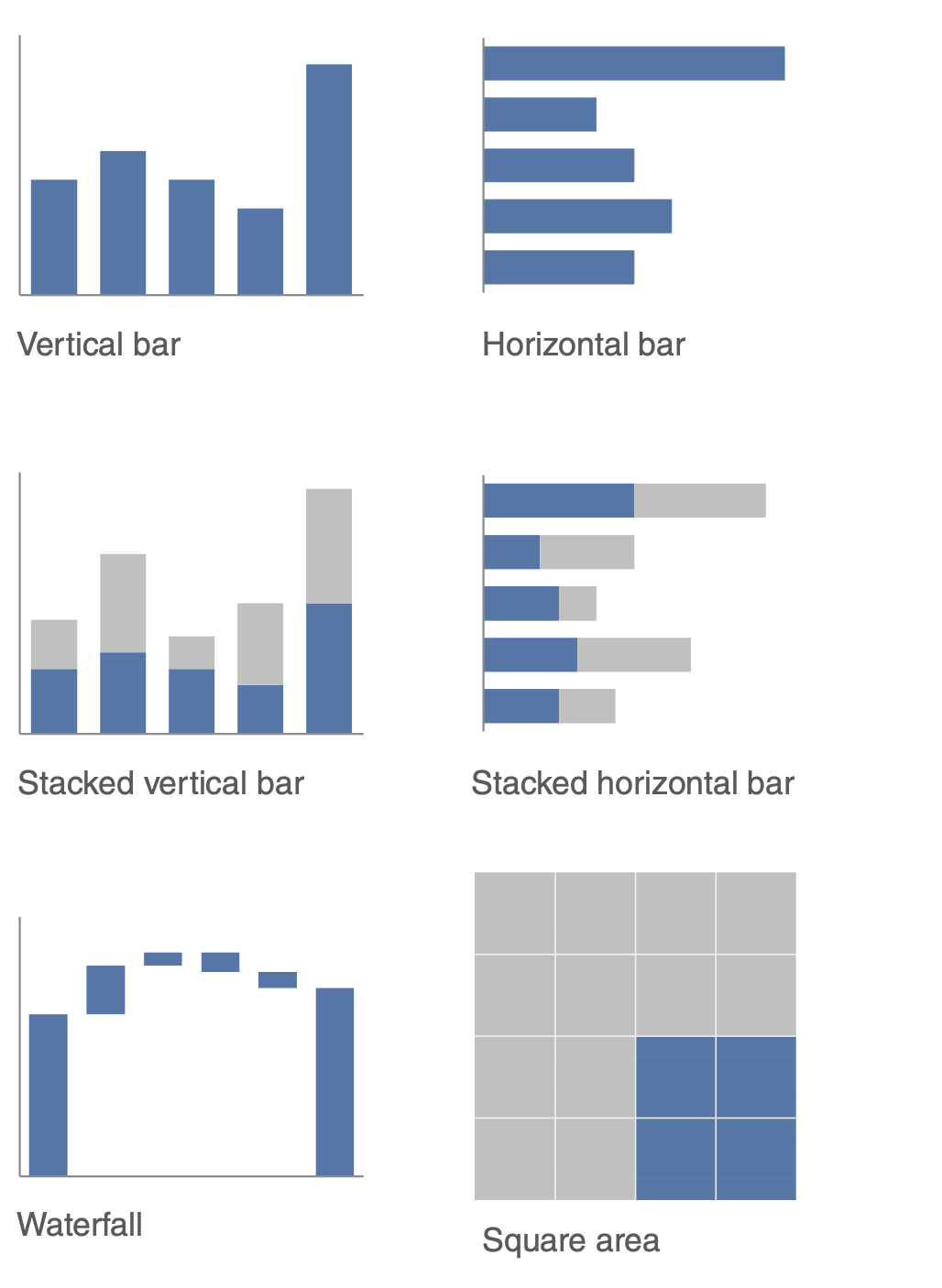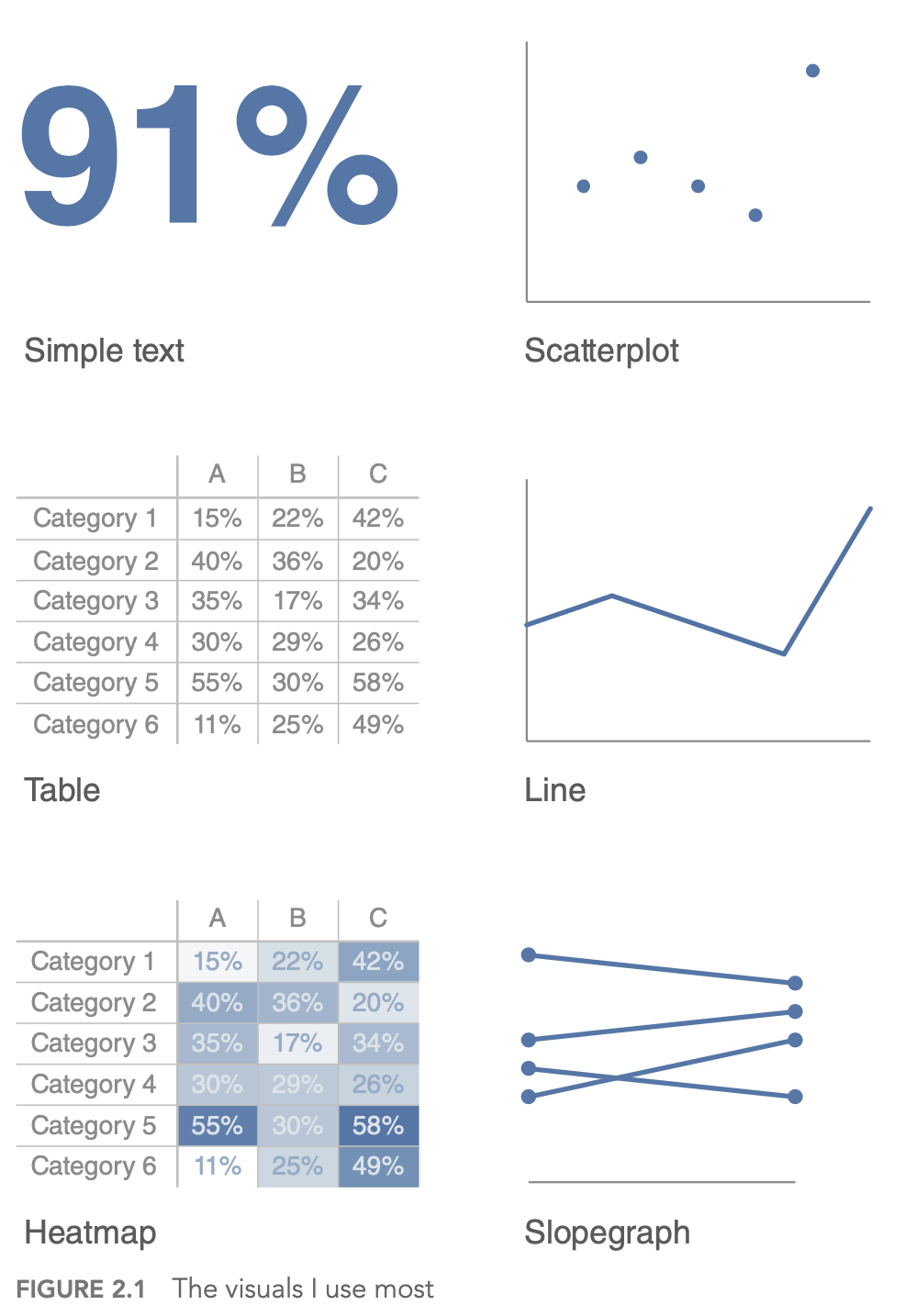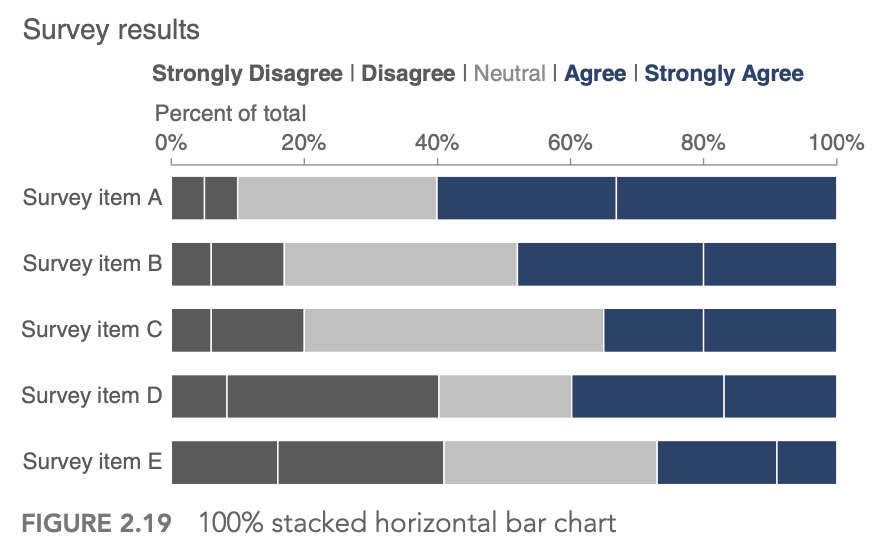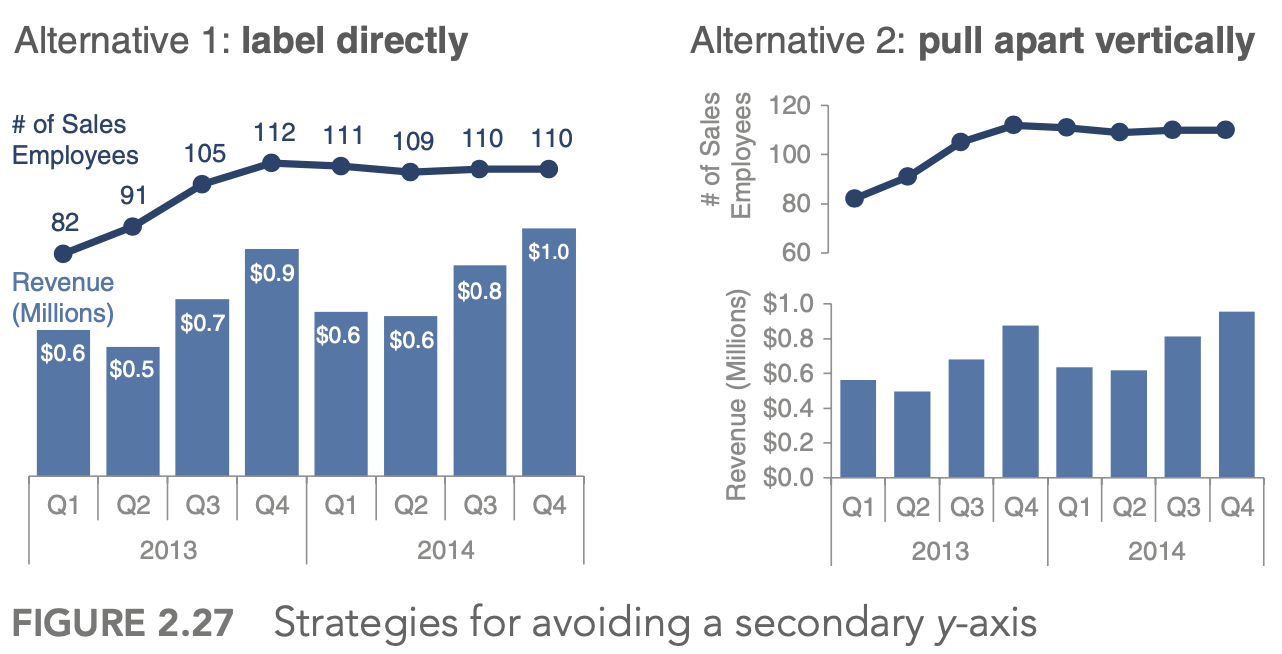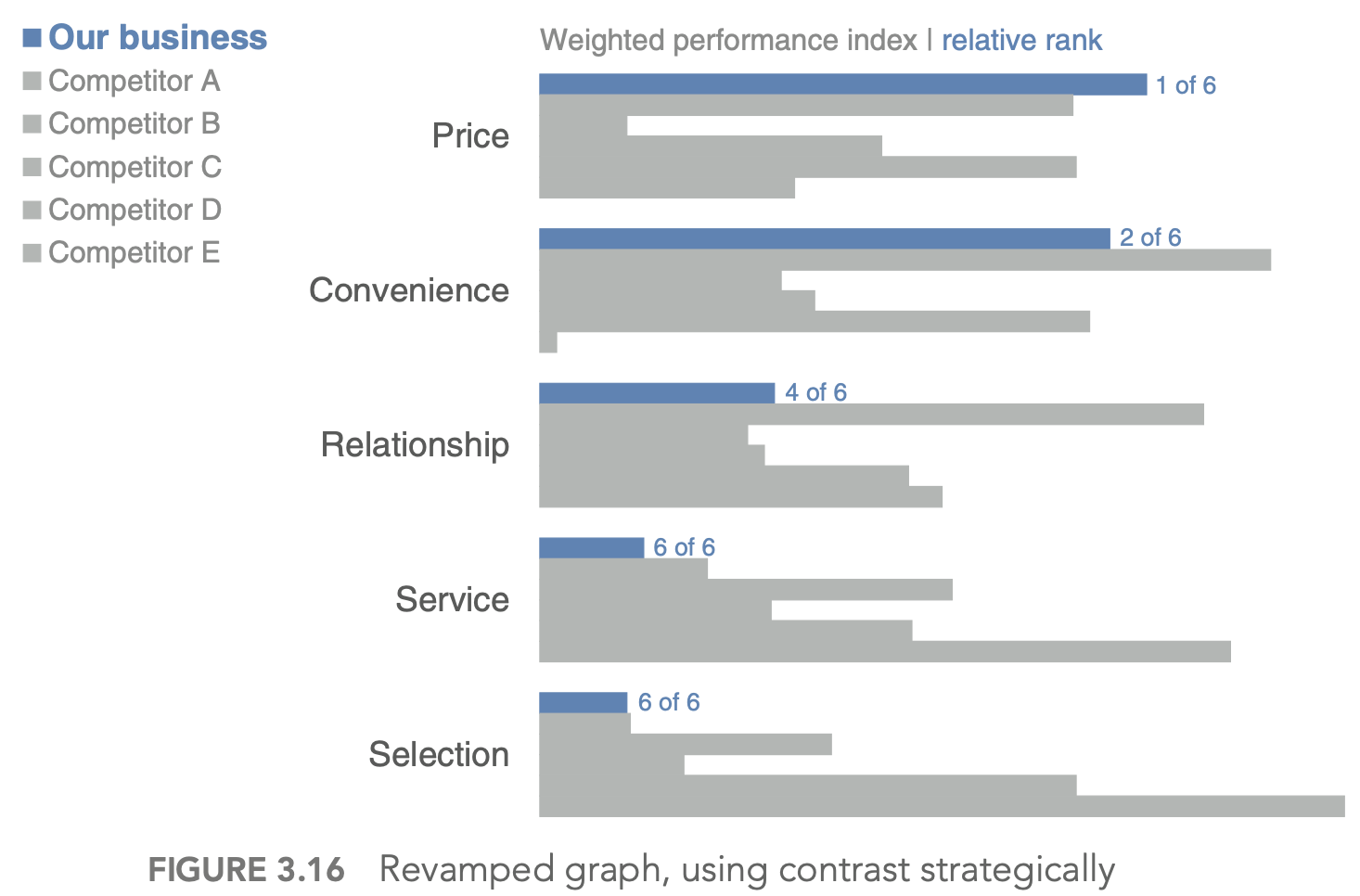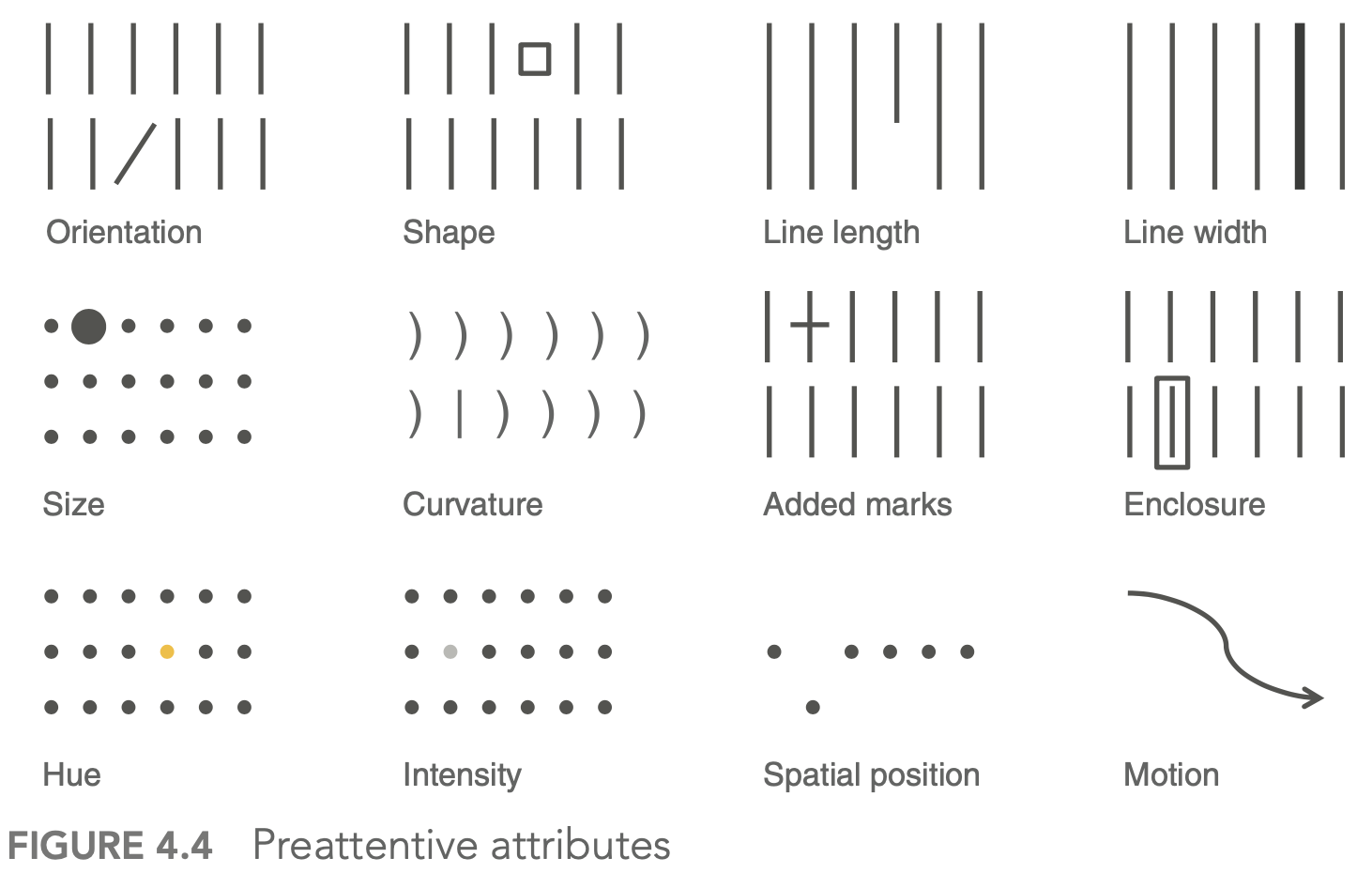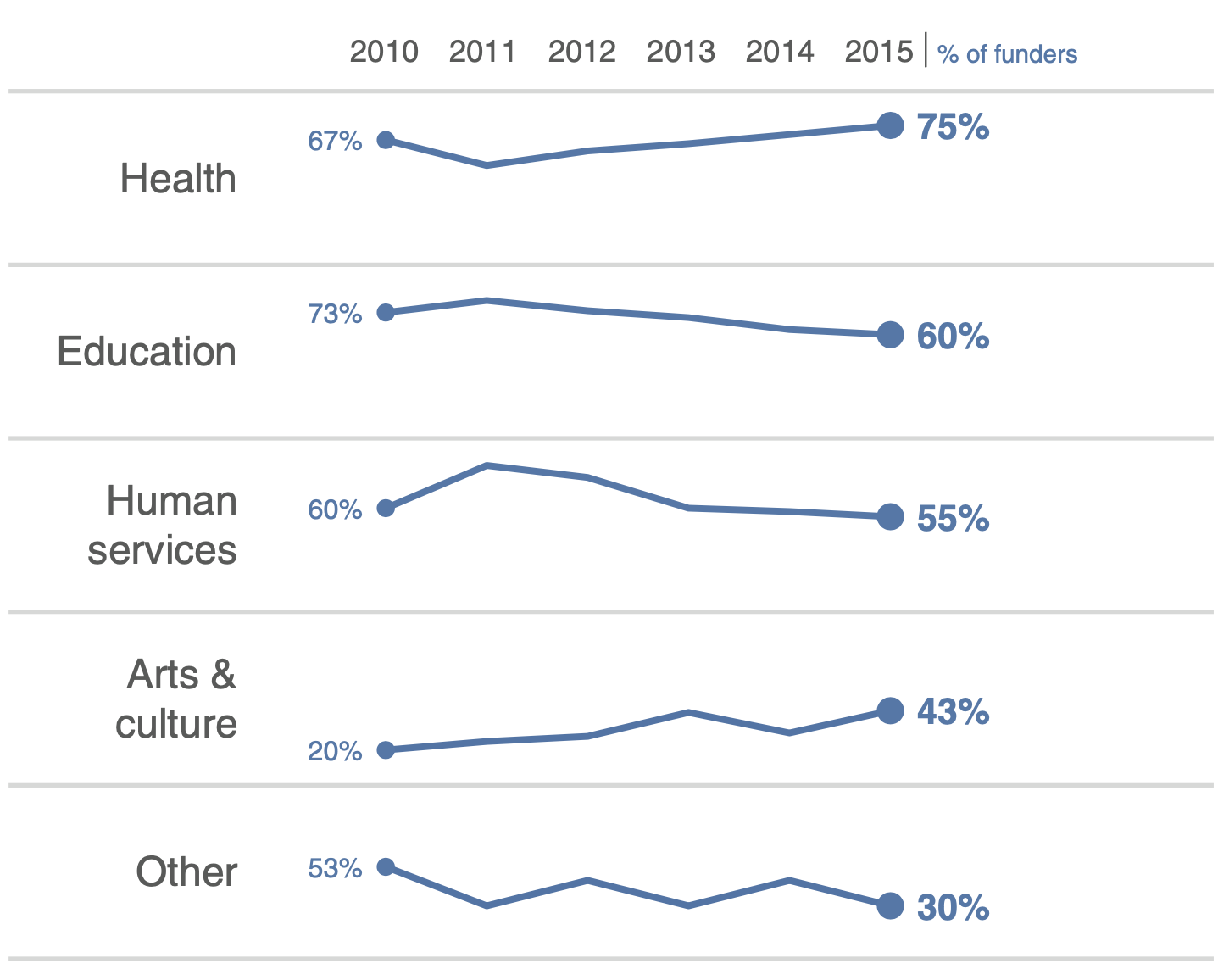• generally avoid pie/donut charts, 3D charts, 2nd y-axes
• tables
• best for when people will actually read off numbers
• minimalist is best
• bar charts should basically always start at 0
• horizontal charts typically easy to read
• on axes, retain things like dollar signs, percent, etc.

## eliminate clutter (3)

• gestalt principles of vision
• proximity - close things are grouped
• similarity - similar things are grouped
• connection - connected things are grouped
• enclosure
• closure
• continuity
• generally good to have titles and such at top-left!
• diagonal lines / text should be avoided
• center-aligned text should be avoided
• label lines directly

## focus attention (4)

• visual hierarchy - outlines what is important

## tell a story / think like a designer (5)

• affordances - aspects that make it obvious how something will be used (e.g. a button affords pushing)
• “You know you’ve achieved perfection, not when you have nothing more to add, but when you have nothing to take away” (Saint‐Exupery, 1943)
• stories have different parts, which include conflict + tension
• beginning - introduce a problem / promise
• middle - what could be
• end - call to action
• horizontal logic - people can just read title slides and get out what they need
• can either convince ppl through conventional rhetoric or through a story

## visual summaries

• numerical summaries
• mean vs. median
• sd vs. iq range
• visual summaries
• histogram
• kernel density plot - Gaussian kernels
• with bandwidth h $K_h(t) = 1/h K(t/h)$
• plots
1. box plot / pie-chart
2. scatter plot / q-q plot
• q-q plot = probability plot - easily check normality
• plot percentiles of a data set against percentiles of a theoretical distr.
• should be straight line if they match
3. transformations = feature engineering
• log/sqrt make long-tail data more centered and more normal
• delta-method - sets comparable bw (wrt variance) after log or sqrt transform: $Var(g(X)) \approx [g’(\mu_X)]^2 Var(X)$ where $\mu_X = E(X)$
• if assumptions don’t work, sometimes we can transform data so they work
• transform x - if residuals generally normal and have constant variance
• corrects nonlinearity - transform y - if relationship generally linear, but non-constant error variance
• stabilizes variance - if both problems, try y first - Box-Cox: Y’ = $Y^l : if : l \neq 0$, else log(Y)
4. least squares
• inversion of pxp matrix ~O(p^3)
• regression effect - things tend to the mean (ex. bball children are shorter)
• in high dims, l2 worked best
5. kernel smoothing + lowess
• can find optimal bandwidth
• nadaraya-watson kernel smoother - locally weighted scatter plot smoothing
• $g_h(x) = \frac{\sum K_h(x_i - x) y_i}{\sum K_h (x_i - x)}$ where h is bandwidth - loess - multiple predictors / lowess - only 1 predictor
• also called local polynomial smoother - locally weighted polynomial
• take a window (span) around a point and fit weighted least squares line to that point
• replace the point with the prediction of the windowed line
• can use local polynomial fits rather than local linear fits
6. silhouette plots - good clusters members are close to each other and far from other clustersf

1. popular graphic method for K selection
2. measure of separation between clusters $s(i) = \frac{b(i) - a(i)}{max(a(i), b(i))}$
3. a(i) - ave dissimilarity of data point i with other points within same cluster
4. b(i) - lowest average dissimilarity of point i to any other cluster
5. good values of k maximize the average silhouette score
7. lack-of-fit test - based on repeated Y values at same X values

# imbalanced data

1. randomly oversample minority class
2. randomly undersample majority class
3. weighting classes in the loss function - more efficient, but requires modifying model code
4. generate synthetic minority class samples
1. smote (chawla et al. 2002) - interpolate betwen points and their nearest neighbors (for minority class) - some heuristics for picking which points to interpolate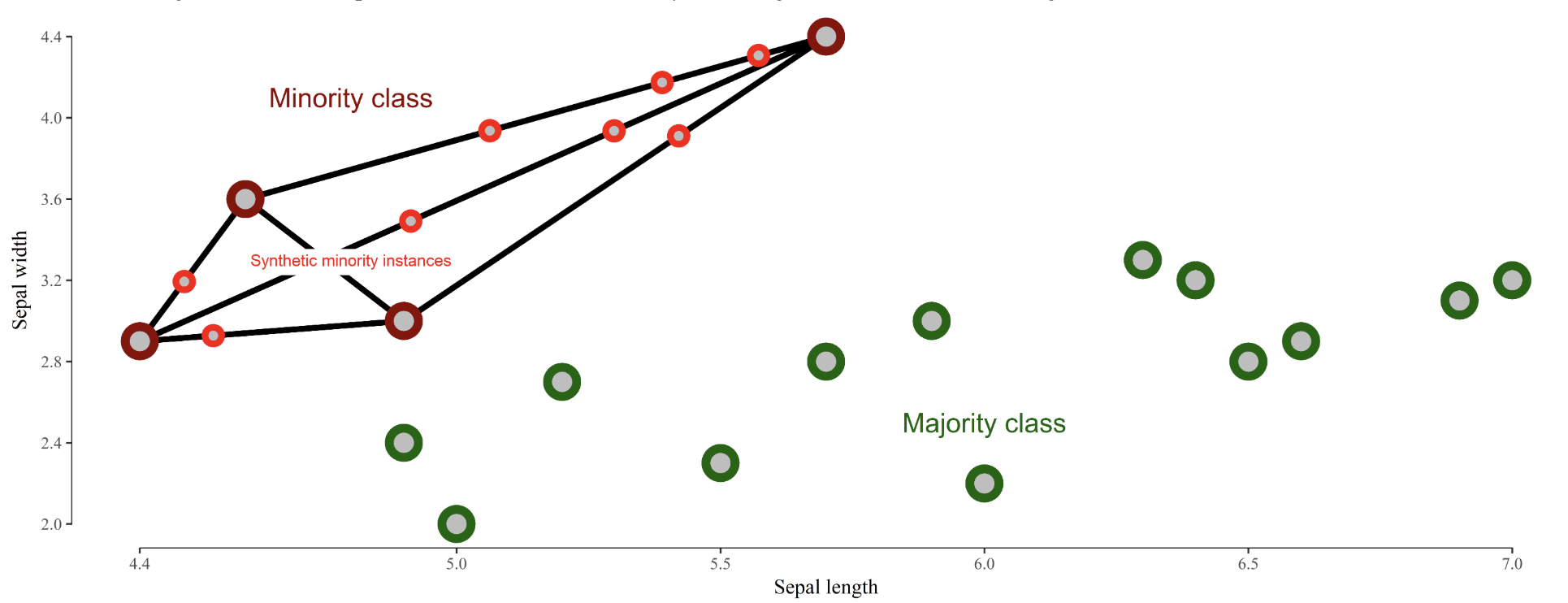1. adasyn (he et al. 2008) - smote, generate more synthetic data for minority examples which are harder to learn (number of samples is proportional to number of nearby samples in a different class)
2. smrt - generate with vae
5. selectively removing majority class samples
1. tomek links (tomek 1976) - selectively remove majority examples until al lminimally distanced nearest-neighbor pairs are of the same class
2. near-miss (zhang & mani 2003) - select samples from the majority class which are close to the minority class. Example: select samples from the majority class for which the average distance of the N closest samples of a minority class is smallest
3. edited nearest neighbors (wilson 1972) - “edit” the dataset by removing samples that don’t agree “enough” with their neighborhood
6. feature selection and extraction
1. minority class samples can be discarded as noise - removing irrelevant features can reduce this risk
2. feature selection - select a subset of features and classify in this space
3. feature extraction - extract new features and classify in this space
4. ideas
1. use majority class to find different low dimensions to investigate
2. in this dim, do density estimation
3. residuals - iteratively reweight these (like in boosting) to improve performance
7. incorporate sampling / class-weighting into ensemble method (e.g. treat different trees differently)
1. ex. undersampling + ensemble learning (e.g. IFME, Becca’s work)
8. algorithmic classifier modifications
9. misc papers
1. enrichment (jegierski & saganowski 2019) - add samples from an external dataset
10. ref
11. imblanced-learn package with several methods for dealing with imbalanced data
12. good blog post
13. Learning from class-imbalanced data: Review of methods and applications (Haixiang et al. 2017)
14. sample majority class w/ density (to get best samples)
15. log-spline - doesn’t scale

# whitening

• get decorrelated features $Z$ from inputs $X$
• $W=$ whitening matrix , selected based on problem goals:
• PCA: Maximal compression of $\mathbf{X}$ in $\mathbf{Z}$
• ZCA: Maximal similarity between $\mathbf{X}$ and $\mathbf{Z}$
• Cholesky: Inducing structure: $\operatorname{Cov}(X, Z)$ is lower-triangular with positive diagonal elements
• $W$ is constrained as to enforce $\Sigma_{Z}=I$

# missing-data imputation

• Missing value imputation: a review and analysis of the literature (lin & tsai 2019)
• Purposeful Variable Selection and Stratification to Impute Missing FAST Data in Trauma Research (fuchs et al. 2014)
• Causal Inference: A Missing Data Perspective (ding & li, 2018)
• different missingness mechanisms (little & rubin, 1987)
• MCAR - missing completely at random - no relationship between the missingness of the data and any values, observed or missing
• MAR - missing at random - propensity of missing values depends on observed data, but not the missing data
• can easily test for this vs MCAR
• MNAR - missing not at random - propensity of missing values depends both on observed and unobserved data
• connections to causal: MCAR is much like randomization, MAR like ignorability (although slightly more general), and MNAR like unmeasured unconfounding
• imputation problem: propensity of missing values depends on the unobserved values themselves (not ignorable)
• simplest approach: drop rows with missing vals
• mean/median imputation
• probabilistic approach
• EM approach, MCMC, GMM, sampling
• matrix completion: low-rank, PCA, SVD
• nearest-neighbor / matching: hot-deck
• (weighted) prediction approaches
• linear regr, LDA, naive bayes, regr. trees, LDA
• can do weighting using something similar to inverse propensities, although less common to check things like covariate balance
• multiple imputation: impute multiple times to get better estimates
• MICE (passes / imputes data multiple times sequentially)
• can perform sensitivity analysis to evaluate the assumption that things are not MNAR
• two standard models for nonignorable missing data are the selection models and the pattern-mixture models (Little and Rubin, 2002, Chapter 15)
• performance evaluation
• acc at finding missing vals

# principles

## breiman

• conversation
• moved sf -> la -> caltech (physics) -> columbia (math) -> berkeley (math)
• info theory + gambling
• CART, ace, and prob book, bagging
• ucla prof., then consultant, then founded stat computing at berkeley
• lots of cool outside activities
• ex. selling ice in mexico
• 2 cultures paper
1. generative - data are generated by a given stochastic model
• stat does this too much and needs to move to 2
• ex. assume y = f(x, noise, parameters)
• validation: goodness-of-fit and residuals
2. predictive - use algorithmic model and data mechanism unknown
• assume nothing about x and y
• ex. generate P(x, y) with neural net
• validation: prediction accuracy
• axioms
3. Occam
4. Rashomon - lots of different good models, which explains best? - ex. rf is not robust at all
5. Bellman - curse of dimensionality - might actually want to increase dimensionality (ex. svms embedded in higher dimension)

## box + tukey

• questions
1. what points are relevant and irrelevant today in both papers?
• relevant
• box
• thoughts on scientific method
• solns should be simple
• necessity for developing experimental design
• flaws (cookbookery, mathematistry)
• tukey
• separating data analysis and stats
• all models have flaws
• no best models
• lots of goold old techniques (e.g. LSR) - irrelevant
• some of the data techniques (I think)
• tukey multiple-response data has been better attacked (graphical models)
2. how do you think the personal traits of Tukey and Box relate to the scientific opinions expressed in their papers?
• probably both pretty critical of the science at the time
• box - great respect for Fisher
• both very curious in different fields of science
3. what is the most valuable msg that you get from each paper?
• box - data analysis is a science
• tukey - models must be useful
• no best models
• find data that is useful
• no best models
• box_79 “science and statistics”
• scientific method - iteration between theory and practice
• learning - discrepancy between theory and practice
• solns should be simple
• fisher - founder of statistics (early 1900s)
• couples math with applications
• data analysis - subiteration between tentative model and tentative analysis
• develops experimental design
• flaws
• cookbookery - forcing all problems into 1 or 2 routine techniques
• mathematistry - development of theory for theory’s sake
• tukey_62 “the future of data analysis”
• general considerations
• data analysis - different from statistics, is a science
• lots of techniques are very old (LS - Gauss, 1803)
• all models have flaws
• no best models
• must teach multiple data analysis methods
• spotty data - lots of irregularly non-constant variability
• could just trim highest and lowest values
• winzorizing - replace suspect values with closest values that aren’t
• must decide when to use new techniques, even when not fully understood
• want some automation
• FUNOP - fulll normal plot
• can be visualized in table
• spotty data in more complex situations

• FUNOR-FUNOM
• multiple-response data
• understudied except for factor analysis
• multiple-response procedures have been modeled upon how early single-response procedures were supposed to have been used, rather than upon how they were in fact used
• factor analysis
1. reduce dimensionality with new coordinates
2. rotate to find meaningful coordinates
• can use multiple regression factors as one factor if they are very correlated
• regression techniques always offer hopes of learning more from less data than do variance-component techniques
• flexibility of attack

• ex. what unit to measure in

## models

• normative - fully interpretable + modelled
• idealized
• probablistic
• ~mechanistic - somewhere in between
• descriptive - based on reality
• empirical

## exaggerated claims

• video by Rob Kass
• concepts are ambiguous and have many mathematical instantiations
• e.g. “central tendency” can be mean or median
• e.g. “information” can be mutual info (reduction in entropy) or squared correlation (reduction in variance)
• e.g. measuring socioeconomic status and controlling for it
• regression “when controlling for another variable” makes causal assumptions
• must make sure that everything that could confound is controlled for
• Idan Segev: “modeling is the lie that reveals the truth”
• picasso: “art is the lie that reveals the truth”
• box: “all models are wrong but some are useful” - statistical pragmatism
• moves from true to useful - less emphasis on truth
• “truth” is contingent on the purposes to which it will be put
• the scientific method aims to provide explanatory models (theories) by collecting and analyzing data, according to protocols, so that
• the data provide info about models
• replication is possible
• the models become increasingly accurate
• scientific knowledge is always uncertain - depends on scientific method